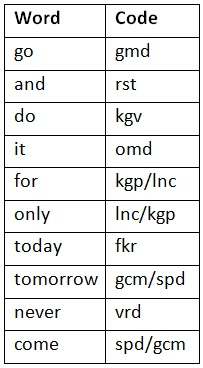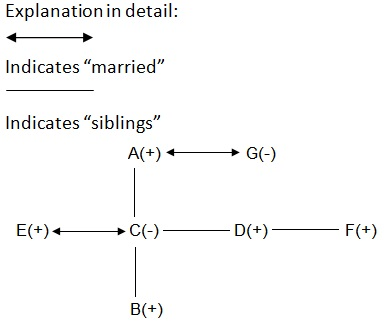# Reasoning Quiz 07Directions (1-5): Study the following information carefully and answer the questions that follow:
In a certain coded language
‘go and do it’ is written as ‘rst, omd, gmd, kgv’
‘do for only today’ is written as ‘lnc, kgv, kgp, fkr’
‘and tomorrow never comes’ is written as ‘vrd, gcm, spd, rst’
‘come today go tomorrow’ is written as ‘spd, fkr, gmd, gem’

Question 1: What is the code of the word “today”?

a. kgp
b. kgv
c. fkr
d. lnc
e. None of the above

c. fkrQuestion 2: Which word is coded as “rst”?

a. go
b. tomorrow
c. never
d. it
e. and

e. andQuestion 3: How is the words ‘go do’ coded?

a. fkr spd
b. gmd kgv
c. spd rst
d. omd gmd
e. gem kgv

b. gmd kgvQuestion 4: Which words are coded as ‘vrd omd’?

a. never it
b. tomorrow do
c. for never
d. it come
e. today only

a. never itQuestion 5: What is the code of the word “come”?

a. lnc
b. kgp
c. gcp
d. spd
e. either (c) or (d)

e. either (c) or (d)Question 6: How many pairs of letters are there in the word “LOCATIONS” which has as many letters between them in the word as in the alphabetical series?

a. Two
b. One
c. Four
d. Three
e. None

b. One
Explanation in detail:
LOCATIONS
NO is the only pair which has as many letters between them in the given word “LOCATIONS” as in the alphabetical series.

Directions (7-8): Study the following information carefully and answer the questions that follow:
Seven persons P, Q, R, S, T, U and V have different heights. U is taller than R. S is taller than U but shorter than P. T is not shorter than Q. Q is not shorter than P. R is not the shortest.
Question 7: If the height of the 2nd shortest person is 227cm. Difference between the heights of R and P is 55cm. What is the probable height of V?

a. 280cm
b. 250cm
c. 234cm
d. 210cm
e. None of the above

d. 210cm
Explanation in detail:
T > Q > P > S > U > R > V

Question 8: Who among the following is taller than U?
I. V
II. T
III. P

a. Only II
b. Only III
c. Only I and II
d. Only I
e. Only II and III

e. Only II and III
Explanation in detail:
T > Q > P > S > U > R > V

Directions (9-10): Study the following information carefully and answer the questions that follow:
There are 7 members A, B, C, D, E, F and G in a family. There are two married couples and three generations in the family.
F is the brother of E’s wife’s brother. A is the father-in-law of E. B is the nephew of D. C is the married daughter of G.
Question 9: How is B relation to F?

a. Son
b. Nephew
c. Father
d. Brother
e. None of the above

b. NephewQuestion 10: How is C related to D?

a. Sister-in-law
b. Daughter
c. Niece
d. Daughter-in-law
e. Sister

e. Sister